## Two long, straight wires are parallel and 10 cm apart. One carries a current of 2.0 A, the other a current of 5.0 A. What is the magnitude a

Question

Two long, straight wires are parallel and 10 cm apart. One carries a current of 2.0 A, the other a current of 5.0 A. What is the magnitude and direction of the force per unit length exerted by the first wire on the second wire if the currents flow in the same direction?

in progress 0
6 months 2021-08-17T07:27:23+00:00 1 Answers 10 views 0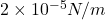and attractive(towards to each other)

Explanation:

We are given that

Distance between wires,d=10 cm=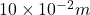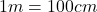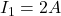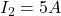We have to find the magnitude and direction of the force per unit length exerted by the first wire on the second wire if the currents flow in the same direction.

We know that magnetic force per unit length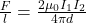Where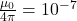Using the formula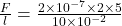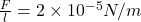When the wires carrying current in same direction then the force between the wires attract to each other.